NEET  >  31 Year NEET Previous Year Questions: System of Particles & Rotational Motion - 3

# 31 Year NEET Previous Year Questions: System of Particles & Rotational Motion - 3

Test Description

## 30 Questions MCQ Test Physics Class 11 | 31 Year NEET Previous Year Questions: System of Particles & Rotational Motion - 3

31 Year NEET Previous Year Questions: System of Particles & Rotational Motion - 3 for NEET 2023 is part of Physics Class 11 preparation. The 31 Year NEET Previous Year Questions: System of Particles & Rotational Motion - 3 questions and answers have been prepared according to the NEET exam syllabus.The 31 Year NEET Previous Year Questions: System of Particles & Rotational Motion - 3 MCQs are made for NEET 2023 Exam. Find important definitions, questions, notes, meanings, examples, exercises, MCQs and online tests for 31 Year NEET Previous Year Questions: System of Particles & Rotational Motion - 3 below.
Solutions of 31 Year NEET Previous Year Questions: System of Particles & Rotational Motion - 3 questions in English are available as part of our Physics Class 11 for NEET & 31 Year NEET Previous Year Questions: System of Particles & Rotational Motion - 3 solutions in Hindi for Physics Class 11 course. Download more important topics, notes, lectures and mock test series for NEET Exam by signing up for free. Attempt 31 Year NEET Previous Year Questions: System of Particles & Rotational Motion - 3 | 30 questions in 60 minutes | Mock test for NEET preparation | Free important questions MCQ to study Physics Class 11 for NEET Exam | Download free PDF with solutions
 1 Crore+ students have signed up on EduRev. Have you?
31 Year NEET Previous Year Questions: System of Particles & Rotational Motion - 3 - Question 1

### A ring of mass m and radius r rotates about an axis passing through its centre and perpendicular to its plane with angular velocity ω. Its kinetic energy is 

Detailed Solution for 31 Year NEET Previous Year Questions: System of Particles & Rotational Motion - 3 - Question 1

Kinetic energy =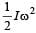and for ring I = mr2

Hence,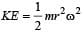31 Year NEET Previous Year Questions: System of Particles & Rotational Motion - 3 - Question 2

### A solid homogeneous sphere of mass M and radius R is moving on a rough horizontal surface, partly rolling and partly sliding. During this kind of motion of the sphere 

Detailed Solution for 31 Year NEET Previous Year Questions: System of Particles & Rotational Motion - 3 - Question 2

Angular momentum a bout the point of contact with the surface includes the angular momentum about the centre. Due to friction linear momentum is conserved.

31 Year NEET Previous Year Questions: System of Particles & Rotational Motion - 3 - Question 3

### A solid cylinder of mass m and radius R rolls down an inclined plane of height h without slipping. The speed of its centre of mass when it reaches the bottom is 

Detailed Solution for 31 Year NEET Previous Year Questions: System of Particles & Rotational Motion - 3 - Question 3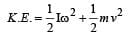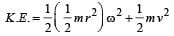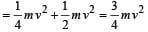Now, gain in K.E. = Loss in P.E.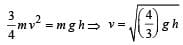31 Year NEET Previous Year Questions: System of Particles & Rotational Motion - 3 - Question 4

A particle of mass  m  is moving in a horizontal circle of radius  R  with uniform speed v. When it moves from one point to a diametrically opposite point, its 

Detailed Solution for 31 Year NEET Previous Year Questions: System of Particles & Rotational Motion - 3 - Question 4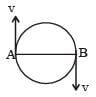At A, momentum = mv

Energy =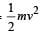At B, momentum = –mv

Energy =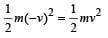Change in momentum = mv - (-mv) = 2mv

Change in energy =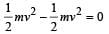31 Year NEET Previous Year Questions: System of Particles & Rotational Motion - 3 - Question 5

Moment of inertia of a uniform circular disc about a diameter is I. Its moment of inertia about an axis ⊥ to its plane and passing through a point on its rim will be 

Detailed Solution for 31 Year NEET Previous Year Questions: System of Particles & Rotational Motion - 3 - Question 5

M.I of uniform circular disc about its diameter = I

According to theorem of perpendiclar axes, M.I. of disc about its axis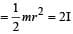Applying theorem of  | | axes,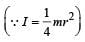M.I of disc about the given axis = 2 I + mr2 = 2 I + 4 I = 6 I

31 Year NEET Previous Year Questions: System of Particles & Rotational Motion - 3 - Question 6

The moment of inertia of a body about a given axis is 1.2 kg m2. Initially, the body is at rest. In order to produce a rotational kinetic energy of 1500 joule, an angular acceleration of 25  radian/sec2 must be applied about that axis for a duration of 

Detailed Solution for 31 Year NEET Previous Year Questions: System of Particles & Rotational Motion - 3 - Question 6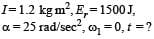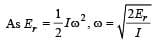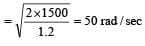From ω2 = ω1 + αt

50 = 0 + 25 t, t = 2 seconds

31 Year NEET Previous Year Questions: System of Particles & Rotational Motion - 3 - Question 7

A fly wheel rotating about a fixed axis has a kinetic energy of 360 joule when its angular speed is 30 radian/sec. The moment of inertia of the wheel about the axis of rotation is 

Detailed Solution for 31 Year NEET Previous Year Questions: System of Particles & Rotational Motion - 3 - Question 7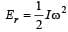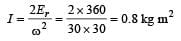31 Year NEET Previous Year Questions: System of Particles & Rotational Motion - 3 - Question 8

A particle of mass m = 5 is moving with a uniform speed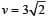in t h e XOY pl ane al o ng the line y = x + 4. The magnitude of the angular momentum of the particle about the origin is 

Detailed Solution for 31 Year NEET Previous Year Questions: System of Particles & Rotational Motion - 3 - Question 8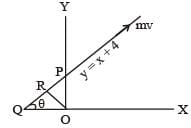y = x + 4 line has been shown in the figure when x = 0, y = 4. So, OP = 4.
The slope of  the line can be obtained by comparing with the equation of the straight line y = mx + c
m = tanθ = 1
⇒ θ = 45°

∠OQP = ∠OPQ = 45°
If we draw a line perpendicular to this line, length of the perpendicular = OR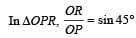⇒ OR = OP sin45°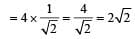Angular momentum of particle going along this line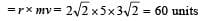31 Year NEET Previous Year Questions: System of Particles & Rotational Motion - 3 - Question 9

If a sphere is rolling , the ratio of the translational energy to total kinetic energy is given by 

Detailed Solution for 31 Year NEET Previous Year Questions: System of Particles & Rotational Motion - 3 - Question 9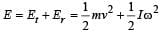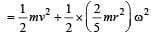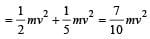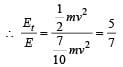31 Year NEET Previous Year Questions: System of Particles & Rotational Motion - 3 - Question 10

The speed of a homogenous solid sphere after rolling down an inclined plane of vertical height h from rest without sliding is 

Detailed Solution for 31 Year NEET Previous Year Questions: System of Particles & Rotational Motion - 3 - Question 10

P.E. = total K.E.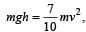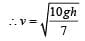31 Year NEET Previous Year Questions: System of Particles & Rotational Motion - 3 - Question 11

A solid  sphere, disc and solid cylinder all of the same mass and made of the same material are allowed to roll down (from rest ) on the inclined plane, then 

Detailed Solution for 31 Year NEET Previous Year Questions: System of Particles & Rotational Motion - 3 - Question 11

For solid  sphere,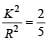For disc and solid cylinder,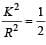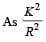for solid sphere is smallest, it takesminimum time to reach the bottom of the incline

31 Year NEET Previous Year Questions: System of Particles & Rotational Motion - 3 - Question 12

In a rectangle ABCD (BC = 2 AB). The moment of inertia is minimum along axis through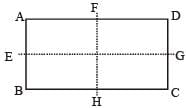Detailed Solution for 31 Year NEET Previous Year Questions: System of Particles & Rotational Motion - 3 - Question 12

The M.I. is minimum about EG because mass distribution is at minimum distance from EG.

31 Year NEET Previous Year Questions: System of Particles & Rotational Motion - 3 - Question 13

Angular momentum is 

Detailed Solution for 31 Year NEET Previous Year Questions: System of Particles & Rotational Motion - 3 - Question 13

Angular momentum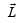is defined as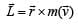So,is  an axial vector..

31 Year NEET Previous Year Questions: System of Particles & Rotational Motion - 3 - Question 14

A spherical ball rolls on a table without slipping.Then the fraction of its total energy associated with rotation is 

Detailed Solution for 31 Year NEET Previous Year Questions: System of Particles & Rotational Motion - 3 - Question 14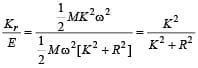Here,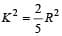31 Year NEET Previous Year Questions: System of Particles & Rotational Motion - 3 - Question 15

A thin uniform circular ring is rolling down an inclined plane of inclination  30° without slipping.
Its linear acceleration along the inclination plane will be 

Detailed Solution for 31 Year NEET Previous Year Questions: System of Particles & Rotational Motion - 3 - Question 15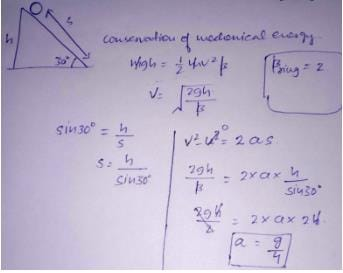31 Year NEET Previous Year Questions: System of Particles & Rotational Motion - 3 - Question 16

The angular speed of an engine wheel making 90 revolutions per minute is 

Detailed Solution for 31 Year NEET Previous Year Questions: System of Particles & Rotational Motion - 3 - Question 16

Number of revolutions made by the engine wheel (n) = 90 per minute.
Angular speed of the engine wheel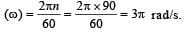31 Year NEET Previous Year Questions: System of Particles & Rotational Motion - 3 - Question 17

Two racing cars of masses m and 4m are moving in circles of radii r and 2r respectively. If their speeds are such that each makes a complete circle in the same time, then the ratio of the angular speeds of the first to the second car is 

Detailed Solution for 31 Year NEET Previous Year Questions: System of Particles & Rotational Motion - 3 - Question 17

We know that both the cars take the same time to complete the circle, therefore ratio of angular speeds of the cars will be 1 : 1.

31 Year NEET Previous Year Questions: System of Particles & Rotational Motion - 3 - Question 18

The ABC is a triangular plate of uniform thickness. The sides are in the ratio shown in the figure. IAB, IBC and ICA are the moments of inertia of the piate about AB, BC and CA respectively. Which one of the following relations is correct ?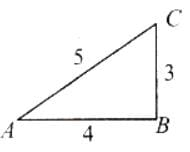Detailed Solution for 31 Year NEET Previous Year Questions: System of Particles & Rotational Motion - 3 - Question 18

The intersection of medians is the centre of mass of the triangle. Since the distances of centre of mass from the sides is related as xBC < xAB < xAC Therefore  IBC  > IAB > IAC or   IBC  > IAB .

31 Year NEET Previous Year Questions: System of Particles & Rotational Motion - 3 - Question 19

If a flywheel makes 120 revolutions/minute, then its angular speed will be 

Detailed Solution for 31 Year NEET Previous Year Questions: System of Particles & Rotational Motion - 3 - Question 19

Angular speed,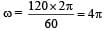rad/sec

31 Year NEET Previous Year Questions: System of Particles & Rotational Motion - 3 - Question 20

The angular momentum of a body with mass (m), moment of inertia (I) and angular velocity (ω) rad/sec is equal to 

Detailed Solution for 31 Year NEET Previous Year Questions: System of Particles & Rotational Motion - 3 - Question 20

Let body contain m1, m2, m3 ........mn masses at distance r1, r2, r3 ........ rn from axis OA.
Angular momentum of body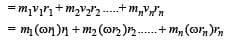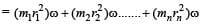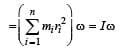31 Year NEET Previous Year Questions: System of Particles & Rotational Motion - 3 - Question 21

A couple produces 

Detailed Solution for 31 Year NEET Previous Year Questions: System of Particles & Rotational Motion - 3 - Question 21

A couple is formed of two equal and opposite forces at some separation; so net force is zero. Hence ,a couple does not produce translatory motion; but it causes change in rotational motion.

31 Year NEET Previous Year Questions: System of Particles & Rotational Motion - 3 - Question 22

The centre of mass of a system of particles does not depend upon 

Detailed Solution for 31 Year NEET Previous Year Questions: System of Particles & Rotational Motion - 3 - Question 22

Centre of mass of system depends upon position and masses of particle. Also, it depends upon relative distance between particles.

31 Year NEET Previous Year Questions: System of Particles & Rotational Motion - 3 - Question 23

In carbon monoxide molecule, the carbon and the oxygen atoms are separated by a distance 1.12 × 10–10 m. The distance of the centre of mass, from the carbon atom is 

Detailed Solution for 31 Year NEET Previous Year Questions: System of Particles & Rotational Motion - 3 - Question 23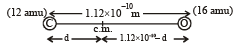From definition of centre of mass.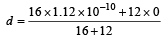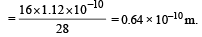31 Year NEET Previous Year Questions: System of Particles & Rotational Motion - 3 - Question 24

A thin circular ring of mass M and radius r is rotating about its axis with a constant angular velocity ω. Two objects each of mass m are attached gently to the opposite ends of a diameter of the ring.The ring will now rotate with an angular velocity 

Detailed Solution for 31 Year NEET Previous Year Questions: System of Particles & Rotational Motion - 3 - Question 24

By the principle of conservation of angular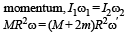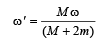31 Year NEET Previous Year Questions: System of Particles & Rotational Motion - 3 - Question 25

O is the centre of an equilateral triangle ABC. F1, F2 and F3 are three forces acting along the sides AB, BC and AC as shown here. What should be the magnitude of F3, so that the total torque about O is zero?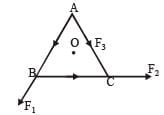Detailed Solution for 31 Year NEET Previous Year Questions: System of Particles & Rotational Motion - 3 - Question 25

Perpendicular distance of each line of action of force from the centre is same, say x. Now taking moments about O,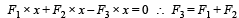31 Year NEET Previous Year Questions: System of Particles & Rotational Motion - 3 - Question 26

A weightless ladder 20 ft long rests against a frictionless wall at an angle of 60º from the horizontal. A 150 pound man is 4 ft from the top of the ladder. A horizontal force is needed to keep it from slipping. Choose the correct magnitude of the force from the following

Detailed Solution for 31 Year NEET Previous Year Questions: System of Particles & Rotational Motion - 3 - Question 26

AB is the ladder, let F be the horizontal force and W is the weigth of man. Let N1 and N2 be normal reactions of ground and wall, respectively. Then for vertical equilibrium W = N1 .....(1)
For horizontal equilibrium, N2 = F .....(2)
Taking moments about A, N2(AB sin60°) – W(AC cos 60°) = 0 ......(3)
Using (2) and AB = 20ft, BC = 4 ft, we get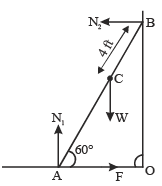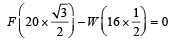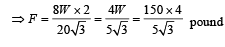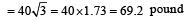31 Year NEET Previous Year Questions: System of Particles & Rotational Motion - 3 - Question 27

Two racing cars of masses m1 and m2 are moving in circles of radii r1 and r2 respectively. Their speeds are such that each makes a complete circle in the same time t. The ratio of the angular speeds of the first to the second car is 

Detailed Solution for 31 Year NEET Previous Year Questions: System of Particles & Rotational Motion - 3 - Question 27

As time taken by both car to complete one revolution is same.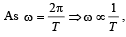as T is same in both

cases. Hence 'ω' will also be same.

31 Year NEET Previous Year Questions: System of Particles & Rotational Motion - 3 - Question 28

The moment of inertia of a disc of mass M and radius R about an axis, which is tangential to the circumference of the disc and parallel to its diameter, is 

Detailed Solution for 31 Year NEET Previous Year Questions: System of Particles & Rotational Motion - 3 - Question 28

Moment of inertia of disc about its diameter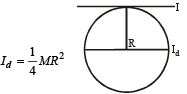MI of disc about a tangent passing through rim and in the plane of disc is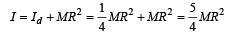31 Year NEET Previous Year Questions: System of Particles & Rotational Motion - 3 - Question 29

There is a flat uniform triangular plate ABC such that AB = 4 cm, BC = 3 cm and angle ABC = 90º.The moment of inertia of the plate about AB, BC and CA as axis is respectively I1, I2 and I3. Which one of the following is true?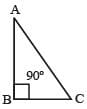Detailed Solution for 31 Year NEET Previous Year Questions: System of Particles & Rotational Motion - 3 - Question 29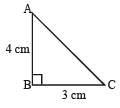Moment of Inertia depend upon mass and distribution of masses as  I = ∑mr2.
Further, as the distance of masses is more , more is the moment of Inertia.
If we choose BC as axis. Distance is maximum. Hence, Moment of Inertia is maximum.

∴ I2 > I1, I2 > I3

31 Year NEET Previous Year Questions: System of Particles & Rotational Motion - 3 - Question 30

A solid cylinder and a hollow cylinder both of the same mass and same external diameter are released from the same height at the same time on an inclined plane. Both roll down without slipping. Which one will reach the bottom first? 

Detailed Solution for 31 Year NEET Previous Year Questions: System of Particles & Rotational Motion - 3 - Question 30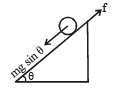Torque, Iα = f. R.
Using Newton's IInd law, mg sinα – f = ma

∵   pure rolling is there, a = Rα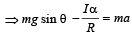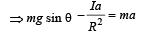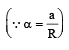or, acceleration,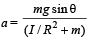Using,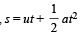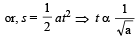t minimum means a should be more. This is possible when I is minimum which is the case for solid cylinder.
Therefore, solid cylinder will reach the bottom first.

## Physics Class 11

127 videos|464 docs|210 tests
 Use Code STAYHOME200 and get INR 200 additional OFF Use Coupon Code
Information about 31 Year NEET Previous Year Questions: System of Particles & Rotational Motion - 3 Page
In this test you can find the Exam questions for 31 Year NEET Previous Year Questions: System of Particles & Rotational Motion - 3 solved & explained in the simplest way possible. Besides giving Questions and answers for 31 Year NEET Previous Year Questions: System of Particles & Rotational Motion - 3, EduRev gives you an ample number of Online tests for practice

## Physics Class 11

127 videos|464 docs|210 tests

### How to Prepare for NEET

Read our guide to prepare for NEET which is created by Toppers & the best Teachers